﻿ 载荷分布对空气静压轴承振动特性的实验Download PDF文章快速检索 高级检索

1. 昆明理工大学 机电工程学院, 昆明 650500;
2. 昆明理工大学 云南省内燃机重点实验室, 昆明 650500

Experiment of load distribution on micro-vibration characteristics of aerostatic bearings
WANG Jiyao1, LONG Wei1, WU Mimi1, ZHAO Na1, BI Yuhua2
1. Faculty of Mechanical and Electrical Engineering, Kunming University of Science and Technology, Kunming 650500, China;
2. Yunnan Province Key Laboratory of Internal Combustion Engines, Kunming University of Science and Technology, Kunming 650500, China
Abstract: To solve the problem of vortex-induced vibration of aerostatic bearings, the disc small hole throttled aerostatic pressure bearing is taken as the research object. Based on the principle of vortex excitation and the theory of oscillatory fluid mechanics, the dynamic characteristics and fluid induced vibration stability of gas films are examined. Analysis of the characteristics of three-dimensional cyclonic vorticity distribution is then conducted using the plane flow function. Finally, the influence of offset load on the micro-vibration characteristics of air bearings is explored by means of numerical simulation and experimental tests. The study reveals that the micro vibration of aerostatic bearings is essentially the coupling between the vortex and the wall caused by unstable flow in the film flow field. Specifically, it is determined by pressure pulsation and vorticity distribution. Load distribution on the bearing surface directly determines the pressure gradient and energy conversion trend in the direction of the film height. The change of gas supply pressure and film thickness directly affects energy loss and conversion form in the process of total energy input and flow in the gas film.
Keywords: aerostatic bearing    vortex-excited vibration    load position    vortic distribution    three-dimensional cyclone

1 理论模型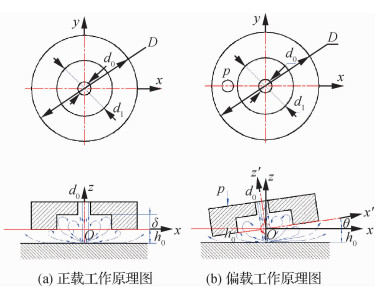图 1 空气静压止推轴承示意图 Fig. 1 Schematic diagram of aerostatic bearing
 $Q = 2\pi \sqrt {{x^2} + {y^2}} {v_{\rm{r}}}$ （1）

 ${v_{\rm{r}}} = \frac{Q}{{2\pi \sqrt {{x^2} + {y^2}} }} = \sqrt {{u^2} + {v^2}}$ （2）

 $\varphi = \pm \frac{Q}{{2\pi }}{\rm{arctan}}\frac{y}{x}\;\:\frac{{{d_0}}}{2} < x < \frac{D}{2},\frac{{{d_0}}}{2} < y < \frac{D}{2}$ （3）

 $u = \left\{ {\begin{array}{*{20}{l}} { - \frac{1}{{2u}} \cdot \frac{{\partial p}}{{\partial x}} \cdot z({h_0} + \delta - z) + \frac{{{u_2} - {u_1}}}{{h + \delta }} \cdot z + {u_1}}&{0 < \sqrt {{x^2} + {y^2}} < \frac{{{d_1}}}{2}}\\ { - \frac{1}{{2u}} \cdot \frac{{\partial p}}{{\partial x}} \cdot z({h_0} - z) + \frac{{{u_2} - {u_1}}}{h} \cdot z + {u_1}}&{\frac{{{d_1}}}{2} < \sqrt {{x^2} + {y^2}} < \frac{D}{2}} \end{array}} \right.$ （4）
 $v = \left\{ {\begin{array}{*{20}{l}} { - \frac{1}{{2u}} \cdot \frac{{\partial p}}{{\partial y}} \cdot z({h_0} + \delta - z) + \frac{{{v_2} - {v_1}}}{{h + \delta }} \cdot z + {v_1}}&{0 < \sqrt {{x^2} + {y^2}} < \frac{{{d_1}}}{2}}\\ { - \frac{1}{{2u}} \cdot \frac{{\partial p}}{{\partial y}} \cdot z({h_0} - z) + \frac{{{v_2} - {v_1}}}{h} \cdot z + {v_1}}&{\frac{{{d_1}}}{2} < \sqrt {{x^2} + {y^2}} < \frac{D}{2}} \end{array}} \right.$ （5）

 $p = {p_{\rm{m}}} \cdot {\rm{exp}}\left( { - 0.693\frac{{{y^2}}}{{{h^2}}}} \right)$ （6）

 $\begin{array}{l} \frac{\partial }{{\partial x}}\left( {{{({h_0} + \delta )}^3}p\frac{{\partial p}}{{\partial x}}} \right) + \frac{\partial }{{\partial y}}\left( {{{({h_0} + \delta )}^3}p\frac{{\partial p}}{{\partial y}}} \right) = \\ {\kern 1pt} {\kern 1pt} {\kern 1pt} {\kern 1pt} {\kern 1pt} {\kern 1pt} {\kern 1pt} {\kern 1pt} {\kern 1pt} {\kern 1pt} {\kern 1pt} {\kern 1pt} {\kern 1pt} {\kern 1pt} {\kern 1pt} {\kern 1pt} {\kern 1pt} {\kern 1pt} {\kern 1pt} {\kern 1pt} {\kern 1pt} {\kern 1pt} {\kern 1pt} {\kern 1pt} {\kern 1pt} {\kern 1pt} {\kern 1pt} {\kern 1pt} {\kern 1pt} \begin{array}{*{20}{l}} {12\mu \frac{\partial }{{\partial t}}(p({h_0} + \delta )) + }\\ {6\mu \left[ {({u_1} + {u_2})\left( {\frac{{\partial (p({h_0} + \delta ))}}{{\partial x}} + } \right.} \right.}\\ {\left. {\left. {({v_1} + {v_2})\frac{{\partial (p({h_0} + \delta ))}}{{\partial y}}} \right)} \right]} \end{array} \end{array}$ （7）

 $\begin{array}{*{20}{c}} {\frac{\partial }{{\partial x}}\left( {h_0^3p\frac{{\partial p}}{{\partial x}}} \right) + \frac{\partial }{{\partial y}}\left( {h_0^3p\frac{{\partial p}}{{\partial y}}} \right) = 12\mu \frac{\partial }{{\partial t}}(p{h_0}) + }\\ {6\mu \left[ {({u_1} + {u_2})\frac{{\partial (p{h_0})}}{{\partial x}} + ({v_1} + {v_2})\frac{{\partial (p{h_0})}}{{\partial y}}} \right]} \end{array}$ （8）

 ${h_{{\rm{eff}}}} = \left\{ {\begin{array}{*{20}{l}} {h + \Delta h}\\ {h - \Delta h} \end{array}} \right.$ （9）

 $\begin{array}{l} \frac{\partial }{{\partial x}}\left( {{{({h_{{\rm{eff}}}})}^3}p\frac{{\partial p}}{{\partial x}}} \right) + \frac{\partial }{{\partial y}}\left( {{{({h_{{\rm{eff}}}})}^3}p\frac{{\partial p}}{{\partial y}}} \right) = \\ {\kern 1pt} {\kern 1pt} {\kern 1pt} {\kern 1pt} {\kern 1pt} {\kern 1pt} {\kern 1pt} {\kern 1pt} {\kern 1pt} {\kern 1pt} {\kern 1pt} {\kern 1pt} {\kern 1pt} {\kern 1pt} {\kern 1pt} {\kern 1pt} {\kern 1pt} {\kern 1pt} \begin{array}{*{20}{l}} {12\mu \frac{\partial }{{\partial t}}(p{h_{{\rm{eff}}}}) + }\\ {6\mu \left[ {({u_1} + {u_2})\frac{{\partial (p{h_{{\rm{eff}}}})}}{{\partial x}} + ({v_1} + {v_2})\frac{{\partial (p{h_{{\rm{eff}}}})}}{{\partial y}}} \right]} \end{array} \end{array}$ （10）

2 实验原理及实验过程 2.1 实验原理和装置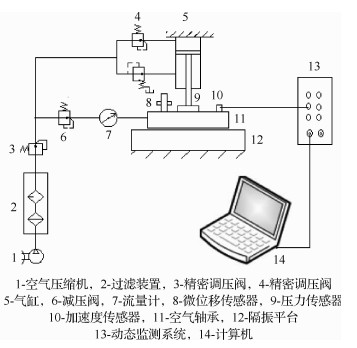图 2 气浮微振动测试原理图 Fig. 2 Schematic of air floatation micro-vibration test

2.2 实验条件及设备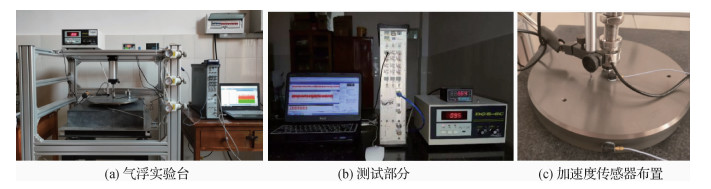图 3 气浮微振动测试装置 Fig. 3 Micro vibration test of air floatation
2.3 实验方法和过程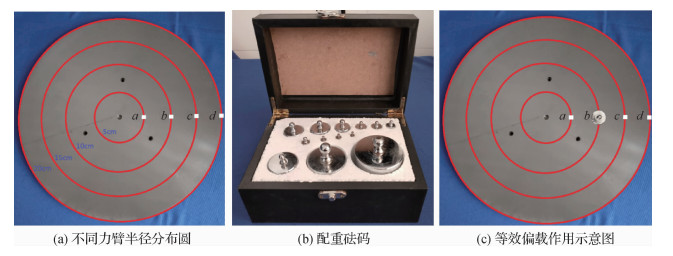图 4 分布载荷测试示意图 Fig. 4 Schematic diagram of partial load moment test

 不同载荷下偏载力矩/kg 载荷力矩/(N·m) a b c d 0.5 0.25 0.5 1 2.5 1 0.5 1 2 5 2 0.75 1.5 3 7.5 5 1 2 4 10
3 结果分析及讨论 3.1 三维微振动验证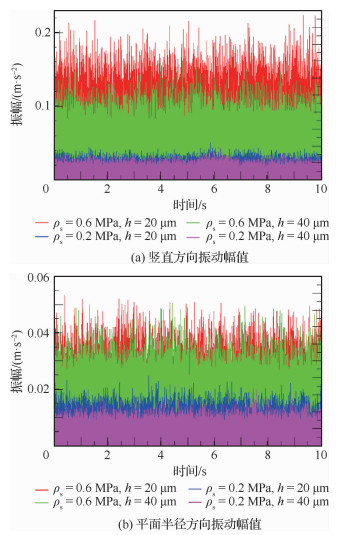图 5 不同供气压力下的时域对比图 Fig. 5 Time domain comparison chart with different gas supply pressure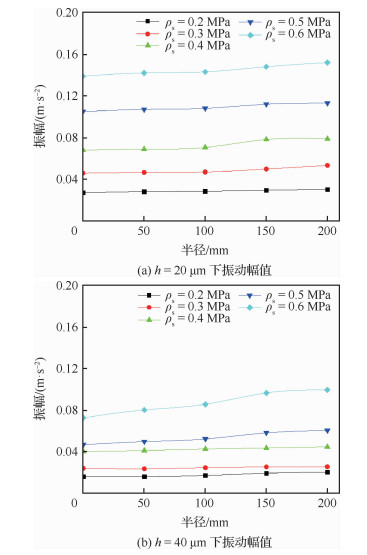图 6 不同载荷分布下的气膜振动幅值 Fig. 6 Vibration amplitudes of gas films with different load distributions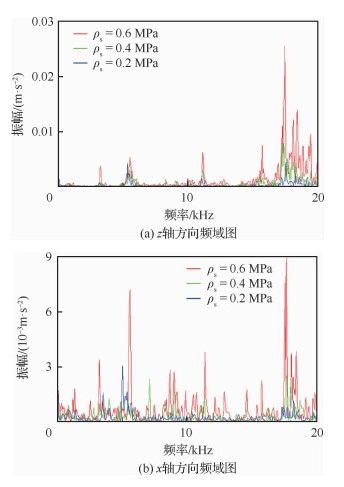图 7 不同供气压力下的气浮支承振动频域信号 Fig. 7 Frequency-domain signals of air floatation at different gas supply pressure
3.2 分布载荷对微振动强度的影响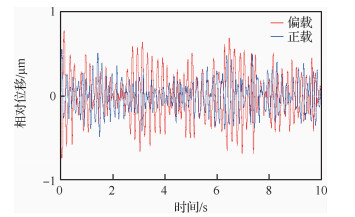图 8 振动时域特性 Fig. 8 Vibration domain comparison chart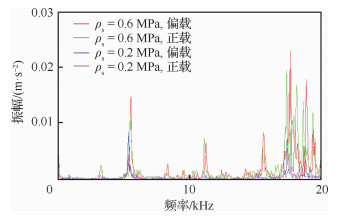图 9 不同供气压力下的的振动频域特性 Fig. 9 Vibration frequency-domain comparison at different gas supply pressure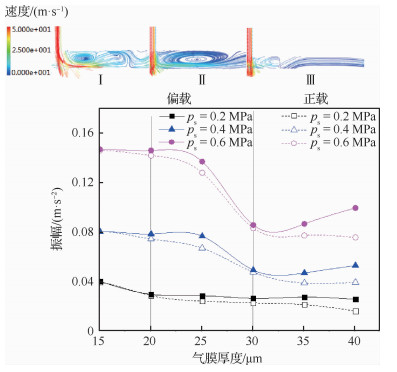图 10 不同供气压力下气膜振动幅值 Fig. 10 Vibration amplitudes of gas film under different gas supply pressure

4 结论

1) 对于空气静压止推轴承，气膜内的涡激振动是由流场内三维气旋形成的涡流形成的。而空气静压轴承的微振动成因，核心是气膜流场流动特性。气膜高压区内局部雷诺数较大，湍流程度较高，带来流场能量的迅速转化和发展。只有当高压区涡动能较低，及涡量分布均匀分散时，形成的涡激效应才能有效减小。反之，则会破坏系统的稳定程度。

2) 轴承表面载荷分布不均匀会增加空气静压轴承的微振动强度。对于小孔节流空气静压轴承而言，相同工况下，随着气膜厚度的增加，振动幅值逐渐减小。在较大气膜下，当供气压力增加到一定时，随着分布载荷偏移位置的增加，振动强度逐渐增强；且高供气压力对微振动影响更敏感。所以在发生偏载下，为减小轴承的振动强度，提高工作稳定性，必须使用较小的供气压力。

3) 轴承气膜内的涡激振动强度会随着供气压力和气膜厚度的增加而增加。相同气供气压力下气体的稠密性降低，导致气膜振动强度降低，而偏置载荷的加入使得气浮轴承气膜流场更为复杂，特别在气膜厚度较大时，气膜的气容量增加，导致偏载对气浮振动强度影响更加明显。

http://dx.doi.org/10.7527/S1000-6893.2020.23679

0

#### 文章信息

WANG Jiyao, LONG Wei, WU Mimi, ZHAO Na, BI Yuhua

Experiment of load distribution on micro-vibration characteristics of aerostatic bearings

Acta Aeronautica et Astronautica Sinica, 2020, 41(8): 223679.
http://dx.doi.org/10.7527/S1000-6893.2020.23679